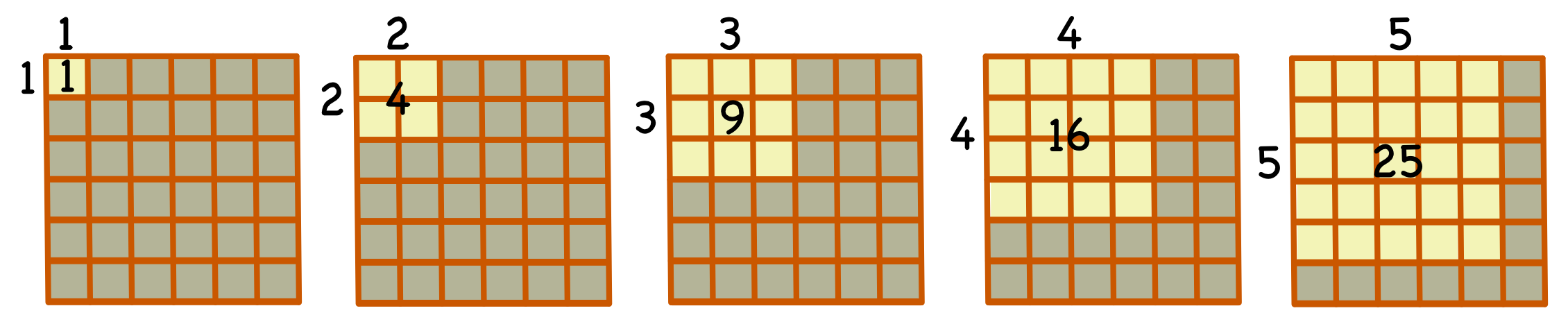Square Sequences

## Square Sequences

A #Square Number# is the result of multiplying an integer by itself. For example, 4 x 4 = 16. The first 5 square numbers are shown below in a diagram form:A shorthand way of writing a square number is to use an #index#: 4 x 4 = 42.

Square numbers can be written as a sequence:

 Term 1 2 3 4 5 ... 12 22 32 42 52 ... Value 1 4 9 16 25 ...

## Example 1

What is the next square number after 81?

81 is 92. The next square number is 102, which is 100.